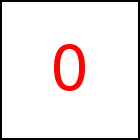# scalarAcceleration Library Method

Short Description: Give Acceleration as Scalar Value

Signature: t.scalarAcceleration (r)
Group: Motion
Class: transition Class

Explicitly sets the acceleration used for a motion with constant acceleration. Please refer to taccgl Transitions for an explanation of motion with constant acceleration. Default acceleration is zero. If r > 0 the acceleration is done in the direction from the starting to the end point of the motion (so the motion speeds up) and if r < 0 the acceleration is done in the direction from the end to the starting point of the motion (so the motion slows down).

As with all motions taccgl™ determines the begin and end velocity automatically. If the acceleration is zero the velocity is fixed throughout the transition and directed from the begin to the end point. If the acceleration in non-zero velocities differ and can even become negative.

Note that either starting or end point or both have to be set (e.g. using from or to). Also note that the acceleration may be set just once using one of the functions acceleration, scalarAcceleration, vBegin and vEnd.

### Examples

 var a=taccgl.actor("pgMiddleColumnTable"); a.to(a.x0,a.y0-400,0).start(); RUN var a=taccgl.actor("pgMiddleColumnTable"); a.to(a.x0,a.y0-400,0) .scalarAcceleration(2).start().cont().start(); RUN var a=taccgl.actor("pgMiddleColumnTable"); a.to(a.x0,a.y0-400,0) .scalarAcceleration(4).start().cont().start(); RUN var a=taccgl.actor("pgMiddleColumnTable"); a.to(a.x0,a.y0-400,0) .scalarAcceleration(8).start().cont().start(); RUN var a=taccgl.actor("pgMiddleColumnTable"); a.to(a.x0,a.y0-400,0) .scalarAcceleration(-2).start().cont().start(); RUN var a=taccgl.actor("pgMiddleColumnTable"); a.to(a.x0,a.y0-400,0) .scalarAcceleration(-8).start().cont().start(); RUN
Next Page:transition.acceleration - Specify Acceleration as Vector
Previous Page: transition.flyToElement - Move object to HTML Element

# Motion

taccgl™ - Canvas Animation Library